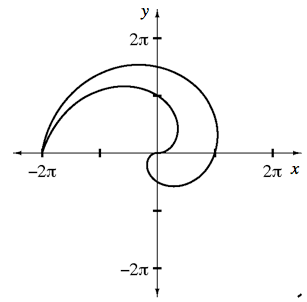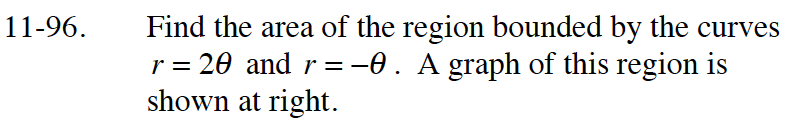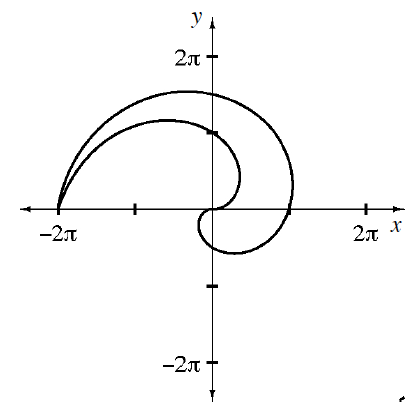### Home > CALC > Chapter 11 > Lesson 11.3.1 > Problem11-96

11-96.
1. Find the area of the region bounded by the curves r = 2θ and r = −θ. A graph of this region is shown below. Homework Help ✎

2. ####Determine the bounds for each curve.$A=\int_0^{2\pi}\frac{1}{2}(-\theta)^2d\theta-\int_0^{\pi}\frac{1}{2}(2\theta)^2d\theta$